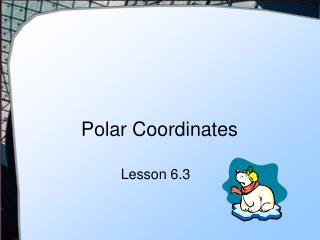# Polar Coordinates - PowerPoint PPT PresentationDownload PresentationPolar Coordinates

Polar Coordinates
Download Presentation## Polar Coordinates

- - - - - - - - - - - - - - - - - - - - - - - - - - - E N D - - - - - - - - - - - - - - - - - - - - - - - - - - -
##### Presentation Transcript

1. Polar Coordinates Lesson 6.3

2. θ r Points on a Plane • Rectangular coordinate system • Represent a point by two distances from the origin • Horizontal dist, Vertical dist • Also possible to represent different ways • Consider using dist from origin, angle formed with positive x-axis (x, y) • (r, θ)

3. Plot Given Polar Coordinates • Locate the following

4. Find Polar Coordinates • What are the coordinates for the given points? • A • A = • B = • C = • D = • B • D • C

5. Converting Polar to Rectangular • Given polar coordinates (r, θ) • Change to rectangular • By trigonometry • x = r cos θy = r sin θ • Try = ( ___, ___ ) • r y θ x

6. Converting Rectangular to Polar • • Given a point (x, y) • Convert to (r, θ) • By Pythagorean theorem r2 = x2 + y2 • By trigonometry • Try this one … for (2, 1) • r = ______ • θ = ______ r y θ x

7. Polar Equations • States a relationship between all the points (r, θ) that satisfy the equation • Example r = 4 sin θ • Resulting values Note: for (r, θ) It is θ (the 2nd element that is the independent variable θ in degrees

8. Graphing Polar Equations • Set Mode on TI calculator • Mode, then Graph => Polar • Note difference of Y= screen

9. Graphing Polar Equations • Also best to keepangles in radians • Enter function in Y= screen

10. Graphing Polar Equations • Set Zoom to Standard, • then Square

11. Try These! • For r = A cos Bθ • Try to determine what affect A and B have • r = 3 sin 2θ • r = 4 cos 3θ • r = 2 + 5 sin 4θ

12. Polar Form Curves • Limaçons • r = B ± A cos θ • r = B ± A sin θ

13. Polar Form Curves • Cardiods • Limaçons in which a = b • r = a (1 ± cos θ) • r = a (1 ± sin θ)

14. Polar Form Curves a • Rose Curves • r = a cos (n θ) • r = a sin (n θ) • If n is odd → n petals • If n is even → 2n petals

15. Polar Form Curves • Lemiscates • r2 = a2 cos 2θ • r2 = a2 sin 2θ

16. Intersection of Polar Curves • Use all tools at your disposal • Find simultaneous solutions of given systems of equations • Symbolically • Use Solve( ) on calculator • Determine whether the pole (the origin) lies on the two graphs • Graph the curves to look for other points of intersection

17. Finding Intersections • Given • Find all intersections

18. Assignment A • Lesson 6.3A • Page 384 • Exercises 3 – 29 odd

19. Area of a Sector of a Circle • Given a circle with radius = r • Sector of the circle with angle = θ • The area of the sector given by θ r

20. Area of a Sector of a Region • Consider a region bounded by r = f(θ) • A small portion (a sector with angle dθ) has area β • dθ α •

21. Area of a Sector of a Region • We use an integral to sum the small pie slices β • r = f(θ) α •

22. Guidelines • Use the calculator to graph the region • Find smallest value θ = a, and largest value θ = b for the points (r, θ) in the region • Sketch a typical circular sector • Label central angle dθ • Express the area of the sector as • Integrate the expression over the limits from a to b

23. The ellipse is traced out by 0 < θ < 2π Find the Area • Given r = 4 + sin θ • Find the area of the region enclosed by the ellipse dθ

24. Areas of Portions of a Region • Given r = 4 sin θ and rays θ = 0, θ = π/3 The angle of the rays specifies the limits of the integration

25. Area of a Single Loop • Consider r = sin 6θ • Note 12 petals • θ goes from 0 to 2π • One loop goes from0 to π/6

26. Area Of Intersection • Note the area that is inside r = 2 sin θand outside r = 1 • Find intersections • Consider sector for a dθ • Must subtract two sectors dθ

27. Assignment B • Lesson 6.3 B • Page 384 • Exercises 31 – 53 odd